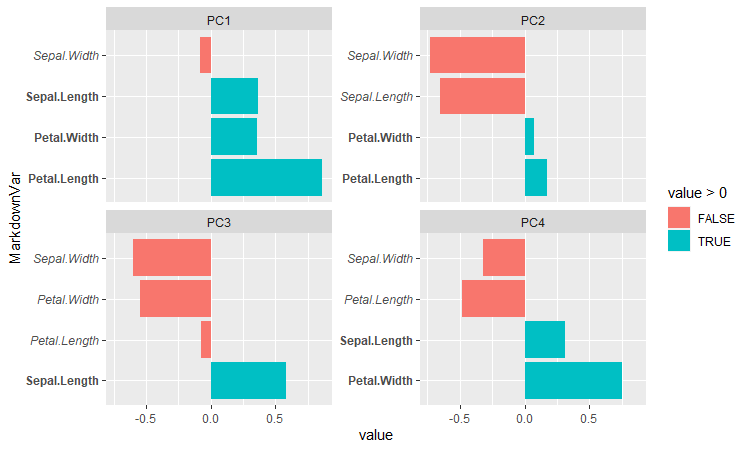[This article was first published on R on Data & The World, and kindly contributed to R-bloggers]. (You can report issue about the content on this page here)
Want to share your content on R-bloggers? click here if you have a blog, or here if you don't.

This is a quick example regarding the `ggtext` package. It’s one of the many packages that extends `ggplot2`, with this one having a focus on adding and formatting text in graphs. The particularly interesting thing for me is that it allows Markdown and other formatting of the labels in a graph.

Let’s throw together a facet plot for the principal components of the `iris` dataset:

```> library(glue)
> library(tidyverse)
> library(ggtext)
> data(iris)
> pca <- prcomp(iris %>% select(matches("Sepal|Petal")))
> transformation <- as.data.frame(pca\$rotation) # prcomp returns matrix, ggplot needs df
> transformation
PC1 PC2 PC3 PC4
Sepal.Length 0.36138659 -0.65658877 0.58202985 0.3154872
Sepal.Width -0.08452251 -0.73016143 -0.59791083 -0.3197231
Petal.Length 0.85667061 0.17337266 -0.07623608 -0.4798390
Petal.Width 0.35828920 0.07548102 -0.54583143 0.7536574```

Since we need to get this to a facet plot, we need to pivot this data into a long format. We need to also preserve the row names, since `pivot_longer()` will just erase them otherwise. This is also when we can add in our Markdown formatting, which will just be italicizing the negative-valued elements of the rotations and bolding the positive parts:

```> transformation <- transformation %>% mutate(Var=row.names(.)) %>%
> pivot_longer(cols=c(PC1, PC2, PC3, PC4)) %>%
> mutate(VarStyle=ifelse(value>0, "**", "*"),
> MarkdownVar=glue("{VarStyle}{Var}{VarStyle}"))```

After that, it’s a simple case of using `ggplot()`. `ggtext` is invoked for the `element_markdown()` function, which is what does the rendering:

```ggplot(transformation, aes(x=MarkdownVar, y=value, fill=value>0)) +
geom_col() + coord_flip() + facet_wrap(~name, scales="free_y") +
theme(axis.text.y=element_markdown())```Or, suppose you wanted to do it in color instead. Markdown itself doesn’t handle this, but `ggtext` does allow for some basic HTML/CSS formatting:

```> transformation <- transformation %>%
> mutate(VarColor=ifelse(value>0, "#00A000", "#A00000"), # hex codes for medium green and red
> ColoredVar=glue("<span style='color:{VarColor}'>{Var}</span>"))
> ggplot(transformation, aes(x=ColoredVar, y=value, fill=value>0)) +
> geom_col() + coord_flip() + facet_wrap(~name, scales="free_y") +
> theme(axis.text.y=element_markdown())```The documentation indicates that the package currently only supports a fairly limited subset of Markdown, HTML, and CSS, but it already looks pretty formidable – the examples include being able to use images as labels for a discrete axis, which is pretty interesting. It feels like it’ll need a lot of `mutate()` and `glue()` calls in the workflow, like I used above, but that’s not much of an issue.